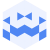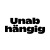Am 14. Nov. 2023 | 19:45 Uhr

# velak gala #128 | Tresor Linz | Nov 14 2023Created at 19. Nov. 2023

142 Ansichten
##### by Tresor Linz

Martini-Windisch
Elizaveta Trukhanova
David Pridal

Tresor Linz - recorded on November 14 2023

<<<<<<<<<<<<<<<<<<<<<<<<<<<<<<<<<<<<<<<<<<<<<<<<<<<<<<<<<
Martini-Windisch
<<<<<<<<<<<<<<<<<<<<<<<<<<<<<<<<<<<<<<<<<<<<<<<<<<<<<<<<<
"Saxophonist Daniele Martini meets sound artist Franziska Windisch, together they dig deep into the realms of acoustic and digital experimentation.
What emerges is density, rarefaction, spatialisation, expansion and contraction of a wide array of sound elements, shapeshifting between melodic and noisy events."

Daniele Martini (saxophone, electronics)
Born in Rome but based in Brussels for a long time, Daniele Martini is a versatilesaxophone player active in different musical areas. With a solid career made of performances all around the globe Martini had the opportunity to perform and record with some amazing musicians of very different fields: Nate Wooley, Tony Allen and Kologbo of Africa ‘70, Jozef Dumoulin, Li Jianhong and many others.
In his own quartet heʼs joined by some remarkable Jazz musicians: Bram De Looze on piano, Manolo Cabras on bass and João Lobo on drums.

Franziska Windisch (laptop, pure data)
Windisch lives and works in Brussels. Her work moves between the areas of performance, text, composition and installation. She composes scores for performative acts which interrogate notions of trace, medium, sound and listening and which she then incorporates in linguistic, sculptural and installative arrangements. Her work has been presented internationally in art spaces and at festivals. Since 2016 she has been teaching with a focus on sound art.

album release https://danielemartini.bandcamp.com/album/well-see-well-see
video excerpt (Smog, Brussels 12/03/2023): https://youtu.be/fRiuIdrXfBI

<<<<<<<<<<<<<<<<<<<<<<<<<<<<<<<<<<<<<<<<<<<<<<<<<<<<<<<<<
Elizaveta Trukhanova
<<<<<<<<<<<<<<<<<<<<<<<<<<<<<<<<<<<<<<<<<<<<<<<<<<<<<<<<<
Elizaveta Trukhanova is a sound artist and musician (harpsichord, organ) currently living in Klosterneuburg. As a child she played the piano and violin and later studied choral conducting in Moscow, church music in Vienna and got a bachelor's degree in harpsichord at Anton Bruckner University in Linz. After recent graduation in electroacoustic and experimental music (ELAK) at University of Music and Applied Arts in Vienna she is now studying Sound Art at Hochschule für Musik und Theater München.
Over the course of a few years Elizaveta has been performing and composing contemporary music. She has taken part in events such as Ars Electronica, leicht über Linz (Linz), Klangspuren Schwaz (Innsbruck), “shut up and listen!” (Vienna) and Muddys Open Stage (Bremen).

Elizaveta is deeply interested in such themes as acceptance, confrontation of the digital and analog world, interaction of nature and technology.

<<<<<<<<<<<<<<<<<<<<<<<<<<<<<<<<<<<<<<<<<<<<<<<<<<<<<<<<<

David Pridal
<<<<<<<<<<<<<<<<<<<<<<<<<<<<<<<<<<<<<<<<<<<<<<<<<<<<<<<<<
Oscillates between the fields of experimental ambient, drone, noise, and sound installations. His livesets are journeys through layers of sound, slowly evolving and modulating from deep reverberating dronescapes, field recordings drifting in to walls of noise to pulsating bassfrequencies, live performed on synths, granular samplers and effect pedals.

David studied media design and painting, lives and works as a illustrator & sound artist in Vienna. He has participated as a musician & sound engineer in events such as the Sonic Territories Festival, the Vienna Improviser Orchestra and FM4 Swoundsound. He has also been a sound designer and live musician for theater productions and dance performances. David plays in various formations as well as solo. Since 2020 he is co-founder of the artist collective Sandkasten Syndikat and Klub Montage, an event series for transdisciplinary improvisation&media art.

<<<<<<<<<<<<<<<<<<<<<<<<<<<<<<<<<<<<<<<<<<<<<<<<<<<<<<<<<
velak
<<<<<<<<<<<<<<<<<<<<<<<<<<<<<<<<<<<<<<<<<<<<<<<<<<<<<<<<<

velak is a non-profit organization/platform for concerts and performances with the focus on electronic, experimental and improvised music, consistently and regularly for almost 20 years. The concert series hosting local and international acts has a nomadic form of organization and is taking place in different settings and spaces around Vienna. Velak pays attention to a balance between established and emerging artists, keeping a collective approach to explore the boundaries of sound, performance and time-based media.

https://velak.klingt.org/

<<<<<<<<<<<<<<<<<<<<<<<<<<<<<<<<<<<<<<<<<<<<<<<<<<<<<<<<<
Tresor Linz
<<<<<<<<<<<<<<<<<<<<<<<<<<<<<<<<<<<<<<<<<<<<<<<<<<<<<<<<<

Raum für Klangkunst und künstlerische Experimente.

Space for sound art and artistic experiments.

##### Share & Embed
Embed this Video